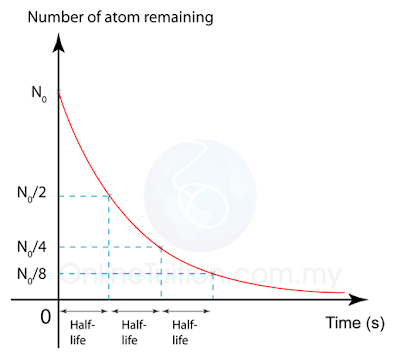# Half-life

### Half-life

1. The half-life ( ${T}_{\frac{1}{2}}$ ) of a quantity subject to exponential decay is the time required for the quantity (mass, number of atom or activity) to decay to half of its initial value.
2.  The decay curve of a radioactive source can be found experimentally - provided the activity of the source decreases over a practicable time period (minutes, hours or days for example).
3. The corrected count-rate is then plotted against time to give the decay curve. The half-life of the source can be found from the curve as indicated above.(Decay Curve)

Example 1
A radioisotope has half-life of 8 hours. Initially, there were 3.6 x 1018 radioisotope atoms in a sample. How much time is taken for the number of atoms of the radioisotope to fall to 4.5 x 1017?

$t=3 T 1 2 t=3(8)=24h$